Passenger car

Passenger car travels 1 km for 2.3 minutes, a truck during a 50 seconds long. How much is the speed of the truck less?

Result

v =  50 km/h

Solution:

x = 1/(2/3/60)
y = 1/(2/3/60+50/3600)
v = x-y

60x = 5400
3600y = 144000
v-x+y = 0

v = 50
x = 90
y = 40

Calculated by our linear equations calculator.

Leave us a comment of this math problem and its solution (i.e. if it is still somewhat unclear...):Be the first to comment!To solve this verbal math problem are needed these knowledge from mathematics:

Do you want to convert length units?

Next similar math problems:

1. FailureDrivers of passenger cars have calculated that at a speed of 60 km/h arrives at destination within 40 minutes. After 20 km refrain five minutes due a technical failure. How fast must go the rest of the way to the finish came at the scheduled time?
2. Freight and passenger car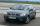The truck starts at 8 pm at 30 km/h. Passenger car starts at 8 pm at 40 km/h. Passenger arrives in the destination city 1 hour and 45 min earlier. What is the distance between the city of departure and destination city?
3. Scale of mapJames travels one kilometer in 12 minutes. The route walked for half an hour measured on the map 5 cm. Calculate how many kilometers James walked for half an hour. Find the scale of the map.
4. Bob traveled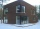Bob traveled 20 meters to Dave's house. He arrived in 3 minutes. What was his average speed for the trip.
5. Runner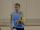Peter ran a speed of 12.4 km / h. After 45 minutes running he had a hiatus. The track is 31 km long. How many kilometers he still to run if he still running at the same speed?
6. WalkersWalker, which makes 120 steps per minute, make distance from point A to point B for 55 minutes. The length of his step is 75cm. For how long does this distance go boy who will do 110 steps 60 cm long in a minute?
7. Pedestrian and the cyclist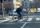Pedestrian and cyclist went against each from the two cities that are distant 40 km. Pedestrian started 30 minutes before the cyclist and walk an average speed of 5 km/h. What speed went cyclist when met after 120 minutes from the start of the pedestrian?
8. Cars 6At 9:00 am two cars started from the same town and traveled at a rate of 35 miles per hour and the other car traveled at a rate of 40 miles per hour. After how many hours will the cars be 30 miles apart?
9. Ping time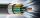Calculate theoretical ping time between Orlando and Shenzhen which is 14102 km distant. Ping time measures the round-trip time for small messages sent from the origin to a destination that is echoed back to the source. The name comes from active sonar term
10. StormSo far, a storm has traveled 35 miles in 1/2 hour in direction straight to observer. If it is currently 5:00 p. M. And the storm is 105 miles away from you, at what time will the storm reach you? Explain how you solved the problem.
11. Mail trainThe speed of mail train is 1370 meter per minute. Express it in miles per hour correct to three significant figure . Given that 1 meter =39.37 inches.
12. Round-tripA woman works at a law firm in city A which is about 50 miles from city B. She must go to the law library in city B to get a document. Find how long it takes her to drive​ round-trip if she averages 40 mph.
13. Aircraft nose downHow long will fall airliner from a height of 10000 m at speed 1,000 km/h?
14. Plan scaleAt what scale is drawn plan of the building, where one side of the building is 45 meters long is on the plan expressed by a straight line 12 mm long.
15. GrandmotherMom walked out to visit her grandmother in a neighboring village 5km away and moved at a speed of 4km/h. An hour later, father drove down the same road at an average speed of 64km/h. 1) How long will take to catch mom die? 2) What is the approximate dis
16. Feet to milesA student runs 2640 feet. If the student runs an additional 7920 feet, how many total miles does the student run?
17. Train expres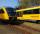At 8.00 strarted from Bratislava to Poprad express Tatry. Cities are distant 340 kilometers. At the same time started from Poprad express Danube to Bratislava. Express Tatry's average speed is 80 km/h, Danube 90 km/h. At what distance from Poprad trains w